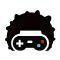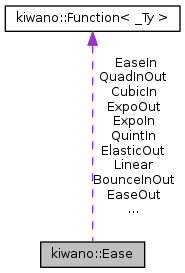Kiwano Engine  v1.2.x
kiwano::Ease结构体 参考

`#include <EaseFunc.h>`

kiwano::Ease 的协作图:[图例]

## 静态 Public 属性

static EaseFunc Linear = math::Linear
线性

static EaseFunc EaseIn = MakeEaseIn(2.f)
由慢变快

static EaseFunc EaseOut = MakeEaseOut(2.f)
由快变慢

static EaseFunc EaseInOut = MakeEaseInOut(2.f)
由慢变快, 再由快变慢

static EaseFunc ExpoIn = math::EaseExponentialIn
由慢变极快

static EaseFunc ExpoOut = math::EaseExponentialOut
由极快变慢

static EaseFunc ExpoInOut = math::EaseExponentialInOut
由慢至极快, 再由极快边慢

static EaseFunc ElasticIn = MakeEaseElasticIn(0.3f)
自起点赋予弹性

static EaseFunc ElasticOut = MakeEaseElasticOut(0.3f)
自终点赋予弹性

static EaseFunc ElasticInOut = MakeEaseElasticInOut(0.3f)
再起点和终点赋予弹性

static EaseFunc BounceIn = math::EaseBounceIn
自起点赋予反弹力

static EaseFunc BounceOut = math::EaseBounceOut
自终点赋予反弹力

static EaseFunc BounceInOut = math::EaseBounceInOut
在起点和终点赋予反弹力

static EaseFunc BackIn = math::EaseBackIn

static EaseFunc BackOut = math::EaseBackOut

static EaseFunc BackInOut = math::EaseBackInOut

static EaseFunc CubicIn = math::EaseCubicIn

static EaseFunc CubicOut = math::EaseCubicOut

static EaseFunc CubicInOut = math::EaseCubicInOut

static EaseFunc QuartIn = math::EaseQuartIn

static EaseFunc QuartOut = math::EaseQuartOut

static EaseFunc QuartInOut = math::EaseQuartInOut

static EaseFunc QuintIn = math::EaseQuintIn

static EaseFunc QuintOut = math::EaseQuintOut

static EaseFunc QuintInOut = math::EaseQuintInOut

static EaseFunc SineIn = math::EaseSineIn

static EaseFunc SineOut = math::EaseSineOut

static EaseFunc SineInOut = math::EaseSineInOut